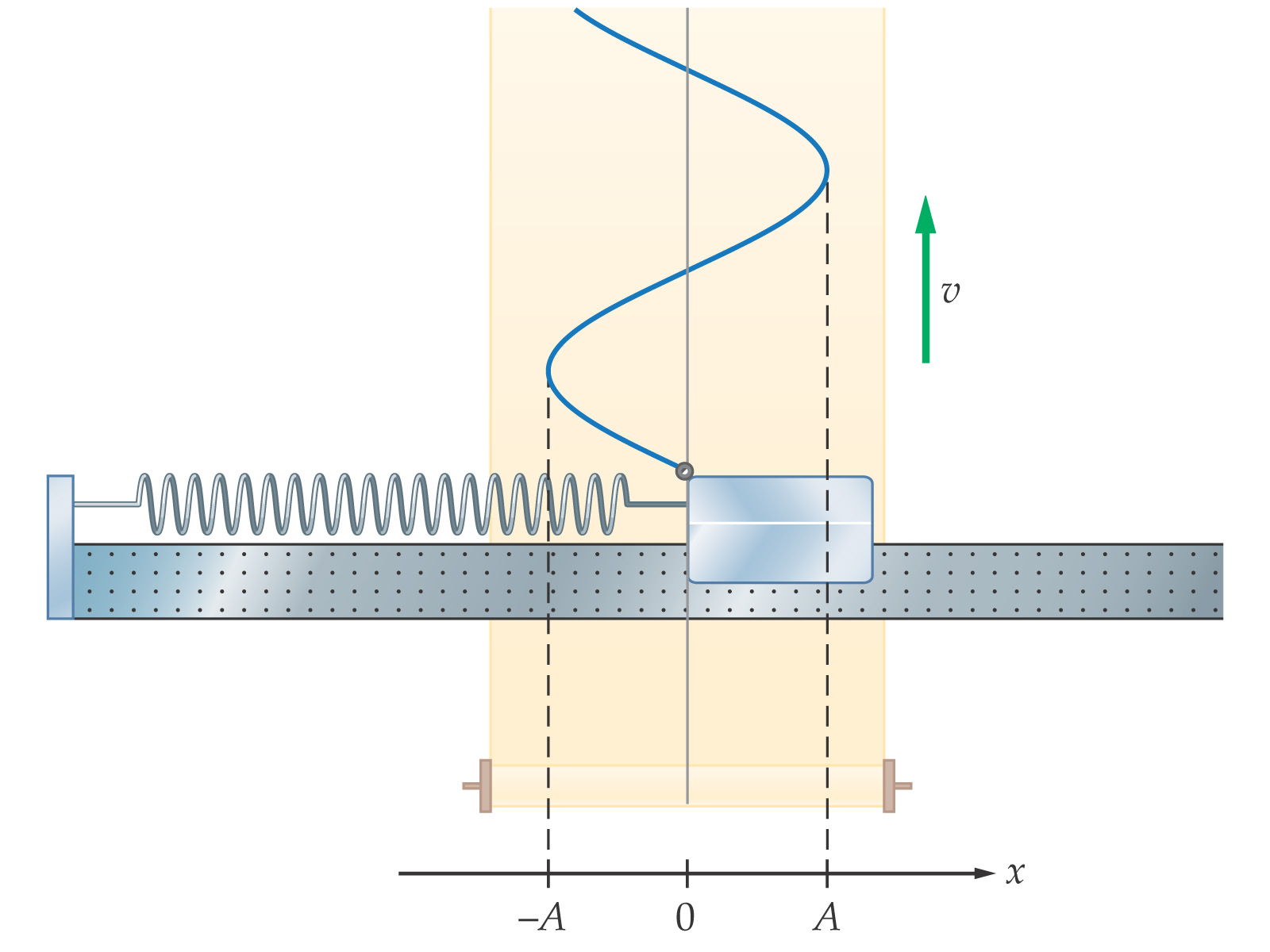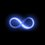# Request: VE: Simple Harmonic MotionGreetings,

I'm currently studying SMH. But, I smh at some of the dumped concepts - and you know how that goes for me. So, I'd like to inquire the following: why does the block-spring system, under no external resistance, behave according to SMH? Or, explain why the following equation applies to the spring-block system:

$y=x_m\cos{\left(\omega t + \phi \right)}$

I understand the entire equation in its description of the behavior of a system that behaves in a SMH. However, one must first prove that a system behaves in a SMH. For example, in the pendulum-gravity system, I know SMH applies since acceleration is described by $g\sin{(\phi)}$ - and thereby integrating twice will give a displacement in a sinusoidal form - hence SMH. But I do not see how that applies at all to the spring-block system - in fact, I do not see how any trigonometric operation could be applied, since angles are not obviously involved.

But we can force them to be involved, can't we? The post below describes my attempts at understanding.

Help is, as always, $\huge{GREATLY}$ appreciated.Note by John M.
5 years, 8 months ago

This discussion board is a place to discuss our Daily Challenges and the math and science related to those challenges. Explanations are more than just a solution — they should explain the steps and thinking strategies that you used to obtain the solution. Comments should further the discussion of math and science.

When posting on Brilliant:

• Use the emojis to react to an explanation, whether you're congratulating a job well done , or just really confused .
• Ask specific questions about the challenge or the steps in somebody's explanation. Well-posed questions can add a lot to the discussion, but posting "I don't understand!" doesn't help anyone.
• Try to contribute something new to the discussion, whether it is an extension, generalization or other idea related to the challenge.
• Stay on topic — we're all here to learn more about math and science, not to hear about your favorite get-rich-quick scheme or current world events.

MarkdownAppears as
*italics* or _italics_ italics
**bold** or __bold__ bold
- bulleted- list
• bulleted
• list
1. numbered2. list
1. numbered
2. list
Note: you must add a full line of space before and after lists for them to show up correctly
paragraph 1paragraph 2

paragraph 1

paragraph 2

[example link](https://brilliant.org)example link
> This is a quote
This is a quote
    # I indented these lines
# 4 spaces, and now they show
# up as a code block.

print "hello world"
# I indented these lines
# 4 spaces, and now they show
# up as a code block.

print "hello world"
MathAppears as
Remember to wrap math in $$ ... $$ or $ ... $ to ensure proper formatting.
2 \times 3 $2 \times 3$
2^{34} $2^{34}$
a_{i-1} $a_{i-1}$
\frac{2}{3} $\frac{2}{3}$
\sqrt{2} $\sqrt{2}$
\sum_{i=1}^3 $\sum_{i=1}^3$
\sin \theta $\sin \theta$
\boxed{123} $\boxed{123}$

## Comments

Sort by:

Top Newest

Well, you're not forcing angles into the picture at all. They emerge naturally from the equation $F=-kx$. If you solve the differential equations involved, you'll obtain one $\sin^{-1}$ term that eventually leads to the result $y=y_0 \cos( \omega t + \phi)$. I have to go to school soon, so I'll just give a broad outline of the procedure.

1. Write $F=-kx$ as $a=-\omega^2 x$
2. Take $a=\dfrac{vdv}{dx}$.
3. Integrate the above using proper limits, keeping in mind that $x$ is the displacement from mean position and that velocity is maximum there.
4. You'll obtain $v$ as a function of $x$.
5. Now write $v$ as $\dfrac{dx}{dt}$.
6. Integrate again, using proper limits, to obtain $x(t)=x_0 \cos (\omega t + \phi)$

So basically, we're not saying that there's a literal angle involved in the physical situation, just that some quantities are oscillating periodically in a sinusoidal manner.

- 5 years, 8 months ago

Log in to reply

DE, huh? Would've never thought of that. Also, doesn't setting $a$ equal to $-\omega^2 x$ presuppose the premise that the motion is harmonic? Then we get back to square one. But I may be missing something here.

Thanks a lot - I'll try to follow the steps and see what I get!

- 5 years, 8 months ago

Log in to reply

No you are not coming back to square one. The fact that simple harmonic motion is defined as a motion in which the acceleration depends upon the displacement. It is the characteristic of a spring that the force applied by it under extension/compression is of that form and hence any body attached to it performs a simple harmonic motion. So, the argument isn't exactly circular. If you still find this confusing, it would be nice if you could just explain the idea of a simple harmonic motion that you have. It would make things easier for both you and me.Thanks!

- 5 years, 8 months ago

Log in to reply

Yes, SMH is defined a motion under influence of a restoring force that is proportional to displacement - but this definition does not, to me, necessarily imply that its amplitude displacement must exhibit a sinusoidal behavior with respect to time. With a pendulum, this makes sense, because acceleration is sinusoidal, and therefore integrating twice will yield a sinusoidal function as well. However, with the block-spring system, apparently you cannot get to sines and cosines without some Differential Equations - which to me is as good conceptually as proving the limit-definition of derivative with deltas and epsilons.

Hence I changed the coordinate system to force the block-spring system into a pendulum so I can apply angular analysis. But that didn't go too well.

Furthermore, a pendulum accelerates due to the force of gravity - which is constant. Yes, the component that matters varies with the angle, but it is nevertheless not directly proportional to displacement - which puts the claim that we can relate it to $F=-kx$ under fire. Yes, for experimental purposes, for small angles, this works. But I'm being mathematical here. Toss all approximations out of the window.

- 5 years, 8 months ago

Log in to reply

Well, I learnt that the fundamental definition of simple harmonic oscillations was a DE of the form $\dfrac{d^2x}{dt^2} = -w^2 x$, where $x$ and $t$ are any quantities, not necessarily position and time. Here, $x$ is varying simple harmonically with $t$. BTW, that screenshot is from Fundamentals of Physics, isn't it? That book is awesome.

- 5 years, 8 months ago

Log in to reply

I don't think he sets $a = - \omega^2x$.

It follows directly from $F = -kx$.

$ma = -kx \implies a = -\frac{k}{m}x \implies a = - \omega^2x$ where $\omega = \sqrt{\frac{k}{m}}$

I'm guessing he brings it to that form to make it easier to manipulate

- 5 years, 8 months ago

Log in to reply

He may not, but my book surely does. And I know the $\omega = \sqrt{\frac{k}{m}}$ relation only as derived from assuming the $a=-\omega^2 x$ relation in the first place - so it is a loaded derivation. Any other way to derive $\omega$?

- 5 years, 8 months ago

Log in to reply

Start with only $F = -kx$. Convert it into $a = \frac{k}{m}x$.

Now perform steps 2 - 6 of Raj's comment.

You should end up with $x(t) = x_0 \cos( \sqrt{\frac{k}{m}}*t + \phi)$. where $\phi = \sin^{-1}{\frac{z}{x_0}}$, $z$ position of block at $t = 0$, and $x_0$ the amplitide.

Now anglular velocity, i.e. $\omega$ will be the derivative of the phase angle w.r.t. time. Phase angle $\theta = \sqrt{\frac{k}{m}}*t + \phi. \implies \frac{d\theta}{dt} = \omega = \sqrt{\frac{k}{m}}$.

- 5 years, 8 months ago

Log in to replyIgnoring the bottom two equations that I slap onto every single paper EVER in order to look cool, the above image describes a trick I've tried to use to force angles into the analysis. So, I have ... sort of... done whatever you see up there. I think I actually converted coordinates from rectangular to polar without realizing. Anyway, I did that so that I can mount a reference point - $O$ - from which a displacement vector pointed at the block will form an angle $\phi$ with the defined vertical that is a line perpendicular to the arc at $x=0$. Notice that here, gravity acts in all directions. Trippy stuff.

Anyway, I did not get far. A few derivations yielded:

$L=\frac{2x_m}{\pi}$

$x=L\phi = \left( \frac{2x_m}{\pi} \right) \phi$

And bunch of other crazy formulas that do nothing but confuse the whole thing. The goal here is to find a sinusoidal expression for either acceleration or velocity - to get displacement - or displacement itself.

You may notice there are a lot of similarities between this new system and that of the pendulum-gravity. However, in this case, the force is variable.

Also, you may notice, that if proven SMH, it also proves any other systems that are influenced by restoring forces linearly proportional to displacement are SMH - because you can model them exactly the same as this.

My book, however, does no more than to say "We can take this Eq in fact to be an alternate definition of SMH." Calling an elephant a donkey doesn't make it one.- 5 years, 8 months ago

Log in to reply

×

Problem Loading...

Note Loading...

Set Loading...